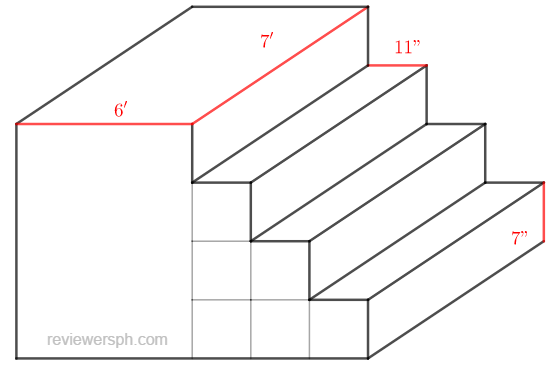### Math Notes

Subjects

#### Solid Geometry Solutions

##### Topics || Problems

A solid concrete porch consists of 3 steps and a landing. The steps have a thread of 11 in arise of 7 in and a length of 7 ft.; the landing is 6 ft by 7ft. How much materials were used in its construction?The materials used in construction is pertaining to the total volume on the porch.

$$V = V_1 + 6V_2$$, where $$V, V_1 ~ and ~ V_2$$ is the total volume, volume of $$6'x7'$$ area and volume of one rectangular parallelepiped step respectively.

$$V_1 = lxwxh = 6x7x\frac{7}{3} = 98$$, $$h = \frac{7x4}{12} = \frac{7}{3}'$$

$$V_2 = \frac{11x7}{144} (7)$$, convert 11 and 7 inches to ft.

$$V_2 = \frac{539}{144}$$

$$V = 98 + (6) (\frac{539}{144})$$

$$V = 120.46 ~ft^3$$ Answer